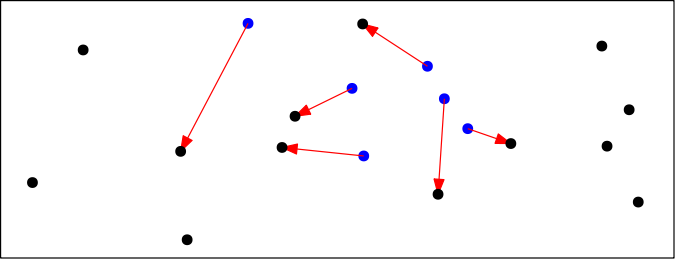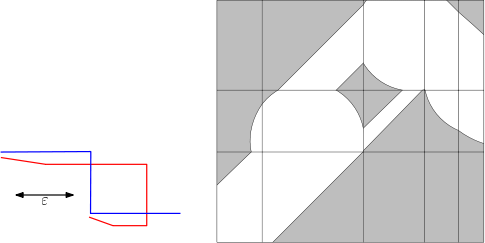Springe direkt zu Inhalt

# Ipelets by Günter Rote

Three ipelets for the extensible drawing editor ipe:

An ipelet is an extension of the extensible drawing editor ipe. See below for installation instructions.

## 1. Point MatchingCompute a minimum-cost one-to-one matching between two point sets. The objective function is the sum of the squared Euclidean distances. The number of matching edges is the smaller of the two point sets (partial matching). The program uses the shortest augmenting path method, of running time O(mn min(m,n)) for two sets of size m and n respectively.

### Usage

Select the two groups of points which you want to match. The cost of the matching is reported in the status line in the lower left corner of ipe's window.

The program can be easily adapted to other objective functions for the edges, like the (unsquared) Euclidean distance, as described near the beginning of the program. (The program can not so easily be adapted to compute a bottleneck objective function, although the algorithm for this problem is actually simpler.)

### Version history

2010-03-25. Version 2. correction in function getpoints. (previous version ignored the point:matrix).

## 2. Free-Space DiagramFor two polygonal curves and a distance threshold ε, the so-called free-space diagram is a tool for computing the Fréchet distance between two curves. It was introduced by Helmut Alt and Michael Godau, Computing the Fréchet distance between two polygonal curves, Internat. J. Comput. Geom. Appl. 5 (1995), 75–91, doi:10.1142/S0218195995000064

### Usage

For the threshold ε, the program uses the length of a line segment, which must be the primary selection. The other two selected items must be two polygonal paths. They can be open or closed paths. They can even consist of several disconnected subpaths, but it is hard to control in which order the subpaths are taken.

In this version of the program, both axes of the free-space diagram are parameterized by arc length. The cells are rectangles and inside each cell, the free space is an ellipse with axes at +/−45°. For two parallel segments, the ellipse degenerates to the region between two parallel lines. (There is also a different version the free-space diagram in which all grid cells are squares.)

### Version history

2010-07-15. Version 2. correction in determining selected objects. (previous version was confused by additional objects, even if they were not selected.)

## 3. Compute the length of a geometric graph (a collection of line segments and polygons)

Select a collection of polygons, polylines or line segments. The applet will compute the total length and display it in the lower left corner, and also print it to the console, in case the program was started by a console command.

You can see the ipelets path in Show configuration in ipe's Help menu. There is a global ipelet directory for the whole installation, and a local ipelet directory for your private installation. (On unix/linux systems, this is usually `\$HOME/.ipe/ipelets`).